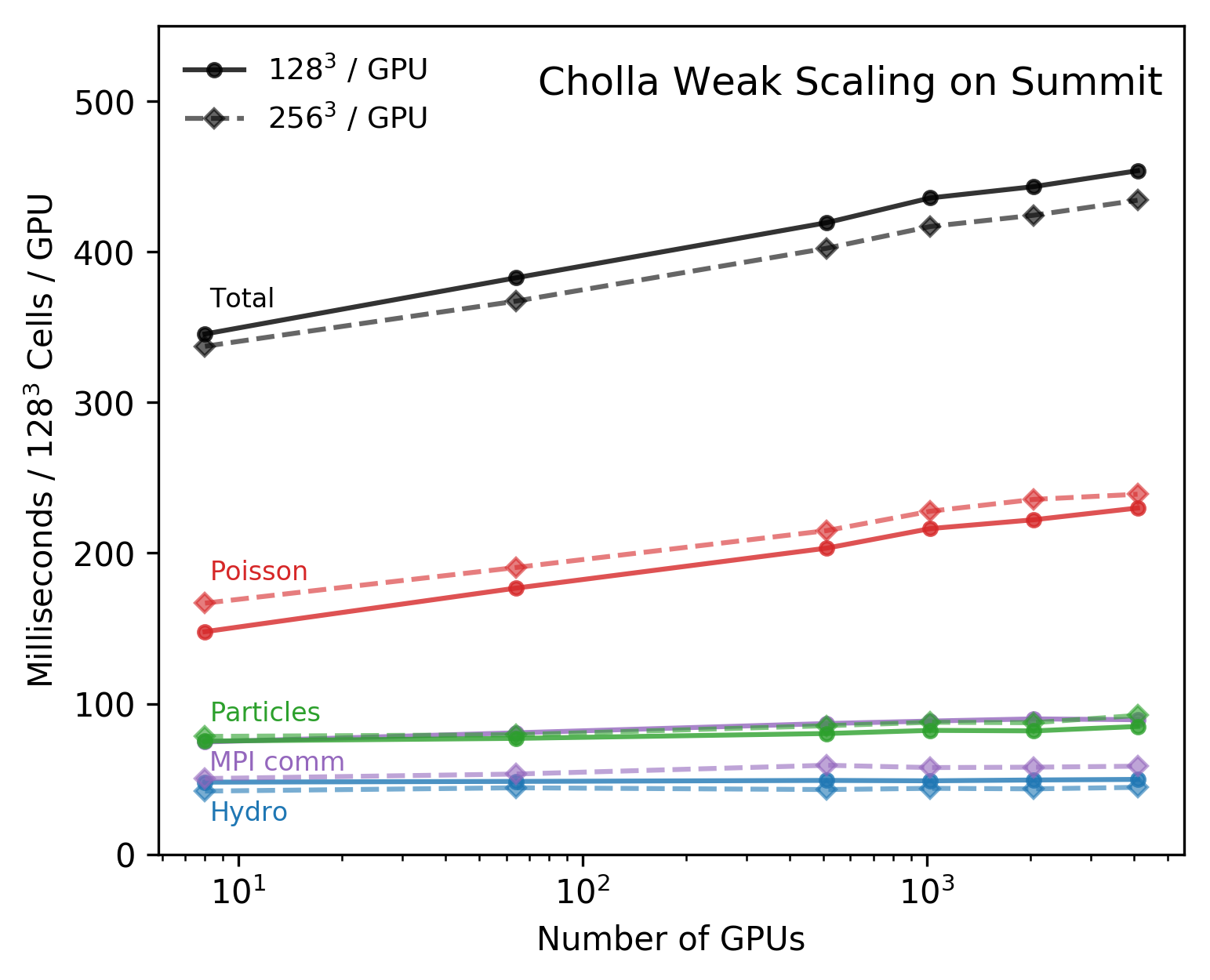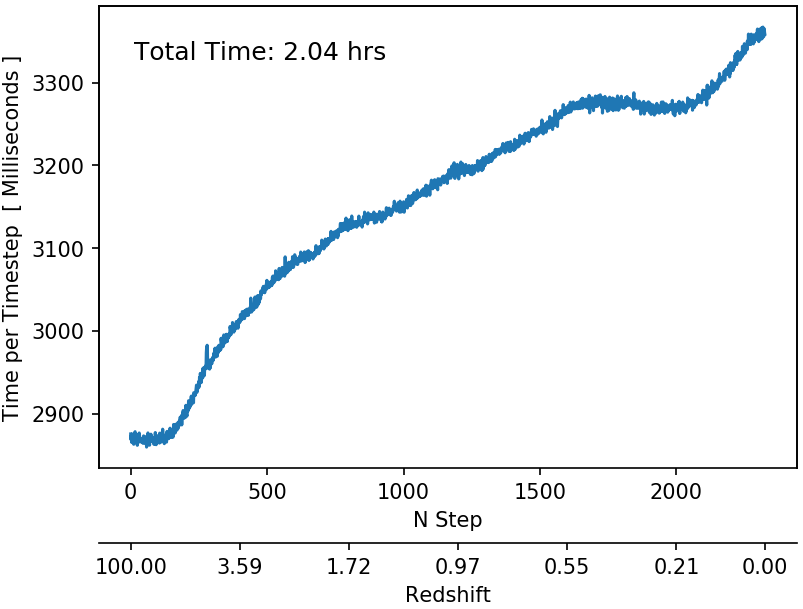## Scaling Test ( Adiabatic )

For this test every GPU evolves a 25Mpc/h sub-volume and every sub-volume has the exact same initial conditions. The times shown in the figure bellow correspond to the time per timestep averaged over the first 30 timesteps of a cosmological simulation starting at $z=100$.For this tests all the GPUs are evolving the same number of particles, because of this, the computational load is balanced across all the processes. In this case the Poisson Solver dominates the calculation time per timestep and increases as the volume of the computation increases due to the $O(n\log{}n)$ nature of the FFTs. I believe that a relaxation method implemented on the GPUs for thee Poisson solver could be faster, but this hasn’t been implemented.

## Cosmological Simulation ( Adiabatic )

On a realistic cosmological simulation the particles distribution becomes less uniform as the simulation progresses, this affects the computational load balance since processes that evolve regions that contain massive halos will have more particles than processes evolving under-dense regions. On a 1024$^3$ cell 50 Mpc/h simulation on 64 GPUs, the timestep at the end of the simulation took ~17% longer than at the beginning of the simulation.The previous figure shows the time per timestep as the simulation progresses. My guess is that this load balance issue will become more pronounced on a intrinsically non-uniform domain.